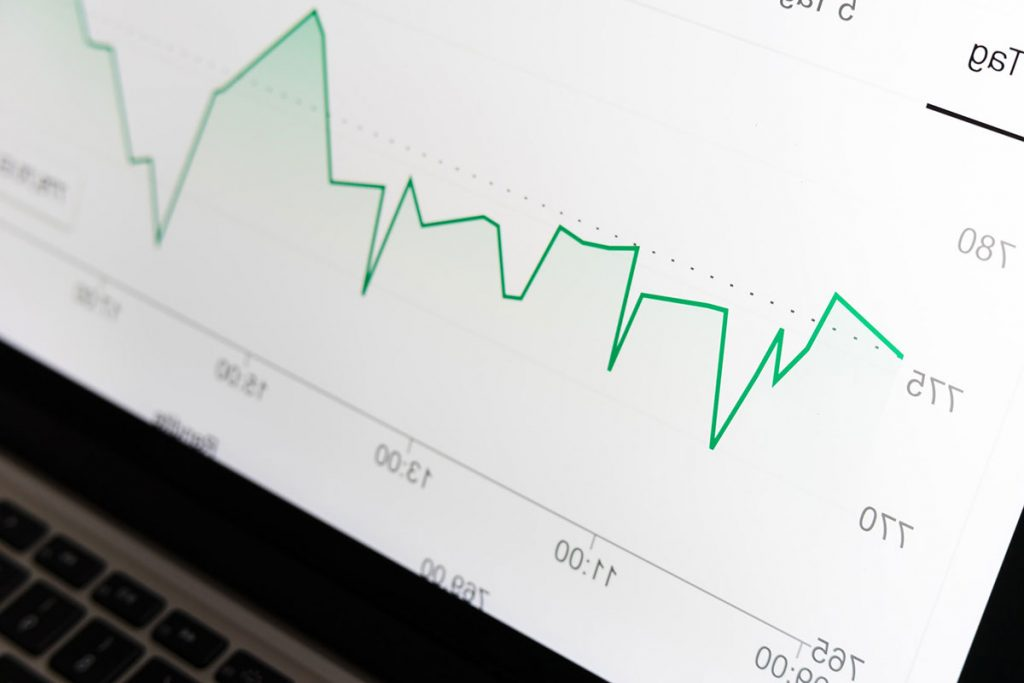## Top 5 Statistical Programming Languages In Demand (2022)

Data scientists require statistical programming languages for a variety of reasons. Using statistics analysis, linear algebra, probability, and calculus, data scientists need tools and coding languages to help them develop algorithms for ML. Linear Regression, Logistic Regression, K Nearest Neighbours, K Means Clustering, and others are some of the most important algorithms. Programming languages that …